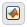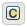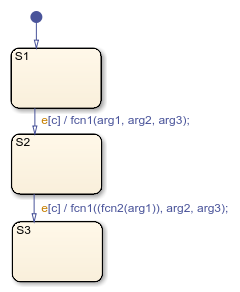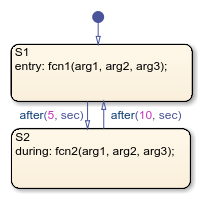## 在 C 语言状态图中调用 C 库函数

•MATLAB® 作为动作语言。

•C 语言作为动作语言。

### 调用 C 库函数

 `abs* **` `acos**` `asin**` `atan**` `atan2**` `ceil**` `cos**` `cosh**` `exp**` `fabs` `floor**` `fmod**` `labs` `ldexp**` `log**` `log10**` `pow**` `rand` `sin**` `sinh**` `sqrt**` `tan**` `tanh**` * Stateflow `abs` 函数有自己的内置功能，超越了与其相当的标准 C 函数的功能。有关详细信息，请参阅调用 abs 函数。 ** 您也可以将对 C 数学库的调用替换为该函数子集的应用程序特定实现。有关详细信息，请参阅用应用程序实现替换数学库函数。

### 调用 abs 函数

• 如果 `x``int32``int64` 类型的整数，则标准 C 函数 `abs` 应用于 `x`，即 `abs(x)`

• 如果 `x``int16``int8` 类型的整数，则将标准 C `abs` 函数应用于转换为 `int32` 类型整数的 `x`，即 `abs((int32)x)`

• 如果 `x``double` 类型的浮点数，则将标准 C 函数 `fabs` 应用于 `x`，即 `fabs(x)`

• 如果 `x``single` 类型的浮点数，则将标准 C 函数 `fabs` 应用于转换为 `double` 类型的浮点数 `x`，即 `fabs((double)x)`

• 如果 `x` 是定点数，则将标准 C 函数 `fabs` 应用于转换为 `double` 类型的定点数，即 `fabs((double) Vx)`，其中 V`x``x` 的实际值。

### 调用 min 和 max 函数

```#define min(x1,x2) ((x1) > (x2) ? (x2):(x1)) #define max(x1,x2) ((x1) > (x2) ? (x1):(x2))```

### 调用自定义 C 代码函数

#### 在图中调用自定义 C 函数的指导原则

• 使用名称、任意参数（置于括号内）以及分号（可选）来定义函数。

• 使用单引号将参数传递给用户编写的函数。例如 `func('string')`

• 一个动作中可以嵌套多个函数调用。

• 动作可以调用返回标量值的函数（对于 MATLAB 函数，为 `double` 类型；对于用户编写的 C 函数，为任何类型）。

#### 编写访问输入向量的自定义 C 函数的指导原则

• 使用 `sizeof` 函数来确定输入向量的长度。

例如，您的自定义函数可以包含使用 `sizeof` 的 for 循环，如下所示：

```for(i=0; i < sizeof(input); i++) { ...... }```
• 如果您的自定义函数多次使用输入向量长度的值，请在函数中包含指定输入向量长度的输入参数。

例如，您可以使用 `input_length` 作为 `sum` 函数的第二个输入，如下所示：

```int sum(double *input, double input_length) ```

您的 `sum` 函数可以包含 for 循环，该循环将遍历输入向量的所有元素：

```for(i=0; i < input_length; i++) { ...... }```

#### 转移动作中的函数调用• `S1` 被激活。

• 发生事件 `e`

• 条件 `c` 为 true。

• 转移目标 `S2` 有效。

`S2``S3` 的转移中的转移动作显示了嵌套在另一个函数调用中的函数调用。

#### 状态动作中的函数调用1. 当到 `S1` 的默认转移发生时，`S1` 被激活。

2. 执行 `entry` 动作，使用指定参数对 `fcn1` 进行函数调用。

3. 在仿真时间经过 5 秒后，`S1` 变为非激活，`S2` 被激活。

4. 执行 `during` 动作，使用指定参数对 `fcn2` 进行函数调用。

5. 在仿真时间经过 10 秒后，`S2` 变为非激活，`S1` 再次被激活。

6. 在仿真结束之前，重复步骤 2 到 5。

#### 按引用传递参数

Stateflow 动作可以按引用（而不是按值）将参数传递给用户编写的函数。特别是，动作可以传递指向值的指针而不是值本身。例如，动作可能包含以下调用：

```f(&x); ```

如果您需要按引用传递某输入项（例如数组），请将该项分配给一个局部数据项并按引用传递该局部数据项。

• 如果 `x` 的数据类型为 `boolean`，则您必须关闭代码生成器选项 使用位集来存储状态配置

• 如果 `x` 是数组且其第一个索引属性设置为 0（请参阅设置数据属性），则必须按如下所示调用函数。

`f(&(x));`

这会将 `x` 的第一个元素的指针传递给函数。

• 如果 `x` 是数组且其第一个索引属性设置为非零数字（例如 1），则必须按以下方式调用函数：

`f(&(x))`;

这会将 `x` 的第一个元素的指针传递给函数。